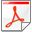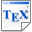[BFK+13] T. Brázdil, V. Forejt, J. Krčál, J. Křetínský and A. Kučera. Continuous-Time Stochastic Games with Time-Bounded Reachability. Information & Computation, 224, pages 46-70, Elsevier. 2013. [pdf] [bib] Downloads:pdf (500 KB)bib Notes: Journal version of [BFK+09] with proofs and improved complexity bounds. Links: [Google] [Google Scholar] [CiteSeer] Abstract. We study continuous-time stochastic games with time-bounded reachability objectives and time-abstract strategies. We show that each vertex in such a game has a value (i.e., an equilibrium probability), and we classify the conditions under which optimal strategies exist. Further, we show how to compute ε-optimal strategies in finite games and provide detailed complexity estimations. Moreover, we show how to compute ε-optimal strategies in infinite games with finite branching and bounded rates where the bound as well as the successors of a given state are effectively computable. Finally, we show how to compute optimal strategies in finite uniform games.